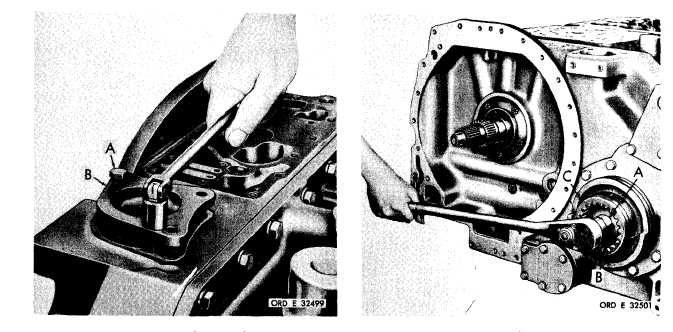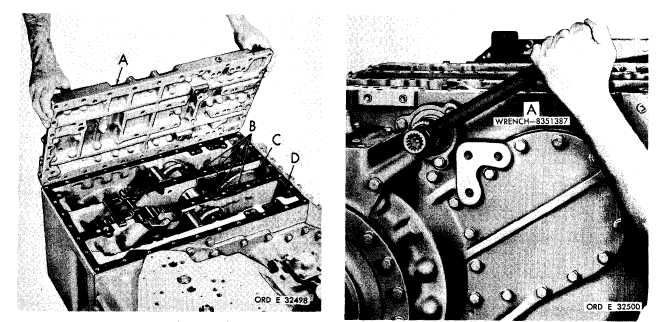Custom SearchC H A P   5,   SEC   I I D I S A S S E M B LY PAR   75,   STEPS  1  5 - 1 8 Figure   79   (Step   15) Remove   transmission   top   cover   plate   (A).   Re- m o v e    f o u r    s e l f - l o c k i n g    b o l t s    ( B ) ,    b a f f l e    ( C) and   gasket   (D). F i g u r e    8 1    ( S t e p    1 7) Using   wrench   (A),   apply   the   transmission   left brake. Figure   80   (Step   16) Using   a   9/16-inch   wrench,   remove   three   bolts (A)   and   lock   washers   retaining   oil   screen   as- sembly   (B).   Using   two   bolts   (A)   as   jacks   crews, loosen   and   remove   screen   assembly   (B). Figure   82   (Step   18) Using   a   1-inch   wrench,   and   with   the   left   brake a p p l i e d , r e m o v e     t h e     l e f t - o u t p u t     s h a f t     s e l f- l o c k i n g    b o l t    ( A ) .    R e m o v e    l o c k    p l a t e    ( B )    a nd coupling    (C). 8 8